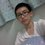# Secret tips for algebra

Hi there,

I have a secret tip for beginners. So, pay attention.

Although the decimal places are all 0 such as 8.00000 can be written as a whole number itself.

Example 1:

Simplify 7.000000 as a whole number.

The decimal places are 0, the solution is easy. So, the answer is 7.

Example 2:

Determine the number either 17.000000009 can be written as a whole number.

This problem is quite a little bit challenging for beginners. But the solution is still very easy for rookies. The solution is to round off the number to the nearest whole number. You will get 17. So, the answer is "Yes".

The problems involving the above is also very easy. The problems can be addition, subtraction, multiplication and so on.

Example 3:

Simplify 19.0000000000 + 6.00000000. The answer must be whole number.

The solution is very easy. Firstly, you need to let all the numbers into whole numbers. You will get 19 + 6. Then, add it up in an easy way. You will get 25. So, the answer is 25.

Example 4:

Solve 91.0800000000 - 60.1000000001. The answer must be whole number.

This problem is quite a little bit challenging for beginners. But is still easy for rookies. Firstly, let all the numbers round off to the nearest whole number. You will get 91 - 60. Next, you can subtract the numbers in an easy way. You will get 31. So, the answer is 31.

Did you get it? If you get it, then try this by yourself. Command if get an answer.

Simplify $6.000000719200^{4} + \sqrt{49.0000000018} - 106.371900000000$. The answer must be whole number.

Waiting for more? Stay tuned for more questions like this on problems.Note by Wesley Har
5 years, 5 months ago

This discussion board is a place to discuss our Daily Challenges and the math and science related to those challenges. Explanations are more than just a solution — they should explain the steps and thinking strategies that you used to obtain the solution. Comments should further the discussion of math and science.

When posting on Brilliant:

• Use the emojis to react to an explanation, whether you're congratulating a job well done , or just really confused .
• Ask specific questions about the challenge or the steps in somebody's explanation. Well-posed questions can add a lot to the discussion, but posting "I don't understand!" doesn't help anyone.
• Try to contribute something new to the discussion, whether it is an extension, generalization or other idea related to the challenge.

MarkdownAppears as
*italics* or _italics_ italics
**bold** or __bold__ bold
- bulleted- list
• bulleted
• list
1. numbered2. list
1. numbered
2. list
Note: you must add a full line of space before and after lists for them to show up correctly
paragraph 1paragraph 2

paragraph 1

paragraph 2

[example link](https://brilliant.org)example link
> This is a quote
This is a quote
    # I indented these lines
# 4 spaces, and now they show
# up as a code block.

print "hello world"
# I indented these lines
# 4 spaces, and now they show
# up as a code block.

print "hello world"
MathAppears as
Remember to wrap math in $$ ... $$ or $ ... $ to ensure proper formatting.
2 \times 3 $2 \times 3$
2^{34} $2^{34}$
a_{i-1} $a_{i-1}$
\frac{2}{3} $\frac{2}{3}$
\sqrt{2} $\sqrt{2}$
\sum_{i=1}^3 $\sum_{i=1}^3$
\sin \theta $\sin \theta$
\boxed{123} $\boxed{123}$

Sort by:

The answer is 1197, but this is only valid when no significant figures involved, or the question do not ask you to specifically round to specific decimal pieces.

- 5 years, 5 months ago